• 在贝叶斯概率理论中，如果后验分布p(θ∣x)p(\theta|x)p(θ∣x)与先验分布p(θ)p(\theta)p(θ)属于同类，则先验分布和后验分布被称为共轭分布，先验分布被称为似然函数的共轭先验。 比如，高斯分布家族在高斯似然...

参考维基百科https://en.wikipedia.org/wiki/Conjugate_prior#Example

共轭先验

在贝叶斯概率理论中，如果后验分布$p(\theta|x)$与先验分布$p(\theta)$属于同类，则先验分布和后验分布被称为共轭分布，先验分布被称为似然函数的共轭先验。
比如，高斯分布家族在高斯似然函数下与其自身共轭 (自共轭)。就是如果似然函数的高斯分布，选择一个高斯先验能够确保后验分布依旧是高斯分布。
具体来说，给定贝叶斯公式$p ( \theta | x ) = \frac { p ( x | \theta ) p ( \theta ) } { \int p ( x | \theta ^ { \prime } ) p \left( \theta ^ { \prime } \right) d \theta ^ { \prime } }$,假定似然函数$p(x|\theta)$已知，问题就是选择什么样的先验分布$p(\theta)$会让后验分布于先验分布具有相同的数学形式。
共轭先验的好处主要在于代数上的方便性，可以直接给出后验分布的封闭形式，否则的话只能数值计算。共轭先验也有助于获得关于似然函数如何更新先验分布的直观印象。所有指数家族的分布都有共轭先验。


展开全文• 其中一类是：共轭先验 共轭先验是指，先验分布与似然函数共轭，即同属于同一分布类。 因为，后验分布=先验分布*似然函数 故如果后验分布也会同先验分布共轭。 而选择共轭先验的原因有两点： 1.共轭先验使得先验...
贝叶斯学派和概率学派的区别之一是特别重视先验信息对inference的影响，而引入先验信息的手段有“贝叶斯原则”（即把先验信息当作均匀分布看）等四大类。
其中一类是：共轭先验
共轭先验是指，先验分布与似然函数共轭，即同属于同一分布类。
因为，后验分布=先验分布*似然函数  故如果后验分布也会同先验分布共轭。
而选择共轭先验的原因有两点：
1.共轭先验使得先验后验的形式相同，这样子更符合人的直观
2.可以形成一个先验链，现在的后验可以做为下一次的先验，如果共轭的话，则形式相同，便可以形成一个链条。

转载于:https://www.cnblogs.com/xiaotu1617234/p/7242807.html
展开全文• 共轭先验 是啥？ 网上找几篇文章，收集与此！ 今天的主要任务是来理解共轭先验以及贝叶斯学习。最近在研究主题模型，里面用到了一些，另外在机器学习中，贝叶斯学习是重要的一个方向，所以有必要学习和掌握。 ...
共轭先验 是啥？
网上找几篇文章，收集与此！

今天的主要任务是来理解共轭先验以及贝叶斯学习。最近在研究主题模型，里面用到了一些，另外在机器学习中，贝叶斯学习是重要的一个方向，所以有必要学习和掌握。

Contents
1.共轭先验分布
2. 贝叶斯学习
3. Beta分布及共轭先验

1.共轭先验分布
什么又是共轭呢？轭的意思是束缚、控制，共轭从字面上理解，则是共同约束，或互相约束。
在贝叶斯概率理论中，如果后验概率P(θ|x)和先验概率p(θ)满足同样的分布律，那么，先验分布和后验分布被叫做共轭分布，同时，先验分布叫做似然函数的共轭先验分布
比如，某观测数据服从概率分布P(θ)时，当观测到新的X数据时，我们一般会遇到如下问题：
可否根据新观测数据X，更新参数θ？
根据新观测数据可以在多大程度上改变参数θ，即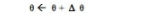当重新估计θ的时候，给出新参数值θ的新概率分布，即P(θ|x)
事实上，根据根据贝叶斯公式可知：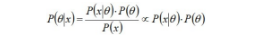其中，P(x|θ)表示以预估θ为参数的x概率分布，可以直接求得，P(θ)是已有原始的θ概率分布。
所以，如果我们选取P(x|θ)的共轭先验作为P(θ)的分布，那么P(x|θ)乘以P(θ)，然后归一化的结果P(θ|x)跟和P(θ)的形式一样。
换句话说，先验分布是P(θ)，后验分布是P(θ|x)，
先验分布跟后验分布同属于一个分布族，故称该分布族是θ的共轭先验分布（族）。
举个例子。投掷一个非均匀硬币，可以使用参数为θ的伯努利模型，θ为硬币为正面的概率，那么结果x的分布形式为：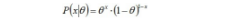其共轭先验为beta分布，具有两个参数α和β，称为超参数（hyperparameters）。且这两个参数决定了θ参数，其Beta分布形式为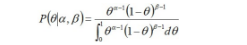然后计算后验概率归一化这个等式后会得到另一个Beta分布，从而证明了Beta分布确实是伯努利分布的共轭先验分布。

2. 贝叶斯学习

首先，我从最简单的硬币投掷开始。现在给你一个硬币，假设有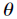的概率为正面朝上，那么有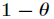的概率是背
面朝上，那么如果在5次投掷过程中，有3次是正面朝上，那么这个最可能是多少呢？

凭着直观感觉，我们可能会认为是3/5，当然这是根据统计规律得到的结论。那么实际上这是一个二项分布，即
重复n次的伯努利实验。由上述所述，很容易知道其概率表示如下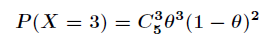我们需要这个概率尽量大，那么最终解得的值为3/5。函数图像如下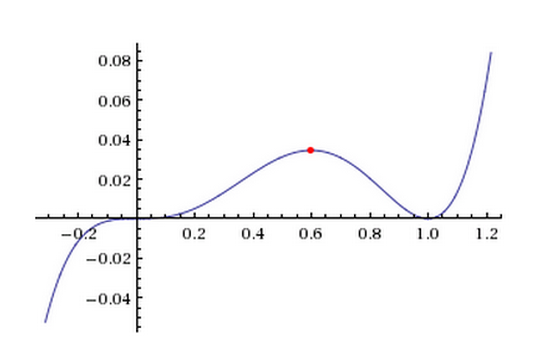但是，我们想象一下，如果在5次投掷过程中，5次都正面朝上，那岂不是得到的估计值是1？ 很明显这种情
况得到的估计值不合理。为了避免这种“黑天鹅事件”的发生，需要将值降低一些才能看似更符合常理，那么
我们只需要乘上另一个小于1的概率值就可以达到了。到了这里贝叶斯公式横空出世！如下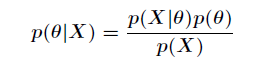其中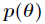叫做先验概率，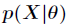叫做似然概率，先验概率是对似然概率的一种补充，如上述的掷硬币。而
后验概率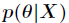正比于似然概率和先验概率的乘积。

3. Beta分布及共轭先验

还是以掷硬币为例，我们已经知道了后验概率正比于似然概率和先验概率的乘积。那么在掷硬币实验中，硬币的
朝向服从伯努利分布，在一系列投掷过程中，假设有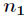次正面朝上，有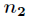次背面朝上，那么似然概率为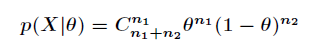现在已经得到了似然概率的形式了，那么如何确定先验概率呢？从理论上来说，任何一个在区间[0, 1]上的分
布函数都符合条件，但是为了更方便地简化计算，最理想的情况就是让先验分布和似然分布有相同的形式，即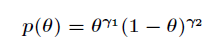如果先验分布是这样的形式，那么计算先验概率和似然概率的乘积就很方便了，只需要将指数相加即可。幸运
的是，有一个很常见的分布恰好满足这个条件，它就是Beta分布。如下其中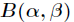是Gamma函数。现在根据先验概率、似然概率和贝叶斯公式来推导后验概率。推导过程如下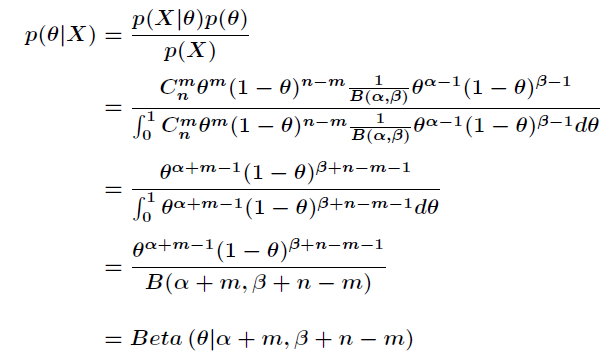在上述中，先验概率叫做似然概率的共轭先验。所谓共轭就是指这两个概率分布具有相同的形式。

最后推荐一些好文章！

一. Beta分布与二项分布的公式原理推导
二. Beta分布与其共轭先验的介绍
三. 多项式分布及Beta分布的期望计算

参考：
http://blog.csdn.net/acdreamers/article/details/45026459http://www.360doc.com/content/16/0428/10/478627_554452907.shtml
转载于:https://www.cnblogs.com/nucdy/p/8484513.html
展开全文• ## 先验概率、后验概率以及共轭先验

万次阅读 多人点赞 2016-05-11 22:24:35
在贝叶斯学派的观点中，先验概率、后验概率以及共轭先验的概念非常重要。而在机器学习中，我们阅读很多资料时也要频繁地跟他们打交道。所以理清这些概念很有必要。本文将通过几个例子详细解释关于先验概率、后验概率...

在贝叶斯学派的观点中，先验概率、后验概率以及共轭分布的概念非常重要。而在机器学习中，我们阅读很多资料时也要频繁地跟他们打交道。所以理清这些概念很有必要。

欢迎关注白马负金羁的博客 http://blog.csdn.net/baimafujinji，为保证公式、图表得以正确显示，强烈建议你从该地址上查看原版博文。本博客主要关注方向包括：数字图像处理、算法设计与分析、数据结构、机器学习、数据挖掘、统计分析方法、自然语言处理。

贝叶斯定理：一个例子

其实我们在之前介绍朴素贝叶斯分类器时就介绍过它，如果你有点忘了，这里就通过一个例子来帮你回忆一下。

假设有一所学校，学生中60%是男生和40%是女生。女生穿裤子与裙子的数量相同；所有男生穿裤子。现在有一个观察者，随机从远处看到一名学生，因为很远，观察者只能看到该学生穿的是裤子，但不能从长相发型等其他方面推断被观察者的性别。那么该学生是女生的概率是多少？

用事件 G$G$ 表示观察到的学生是女生，用事件 T$T$ 表示观察到的学生穿裤子。于是，现在要计算的是条件概率 P(G|T)$P(G|T)$ ，我们需要知道：

P(G)$P(G)$ 表示一个学生是女生的概率。由于观察者随机看到一名学生，意味着所有的学生都可能被看到，女生在全体学生中的占比是 40% ，所以概率是  P(G)=0.4$P(G)=0.4$ 。注意，这是在没有任何其他信息下的概率。这也就是先验概率。后面我们还会详细讨论。
P(B)$P(B)$ 是学生不是女生的概率，也就是学生是男生的概率，这同样也是指在没有其他任何信息的情况下，学生是男生的先验概率。 B$B$ 事件是 G$G$ 事件的互补的事件，于是易得 P(B)=0.6$P(B)=0.6$ 。
P(T|G)$P(T|G)$ 是在女生中穿裤子的概率，根据题目描述，女生穿裙子和穿裤子各占一半，所以 P(T|G)=0.5$P(T|G)=0.5$ 。这也就是在给定 G$G$ 的条件下，T$T$ 事件的概率。
P(T|B)$P(T|B)$ 是在男生中穿裤子的概率，这个值是1。
P(T)$P(T)$ 是学生穿裤子的概率，即任意选一个学生，在没有其他信息的情况下，该名学生穿裤子的概率。根据全概率公式 P(T)=∑ni=1P(T|Ai)P(Ai)=P(T|G)P(G)+P(T|B)P(B)$P(T)=\sum _{i=1}^nP(T|A_i)P(A_i)=P(T|G)P(G)+P(T|B)P(B)$ ，计算得到 P(T)=0.5×0.4+1×0.6=0.8$P(T)=0.5×0.4+1×0.6=0.8$。

根据贝叶斯公式
P(Ai|T)=P(T|Ai)P(Ai)∑ni=1P(T|Ai)P(Ai)=P(T|Ai)P(Ai)P(T)P(A_i|T)=\frac{P(T|A_i)P(A_i)}{\sum _{i=1}^nP(T|A_i)P(A_i)}=\frac{P(T|A_i)P(A_i)}{P(T)}
基于以上所有信息，如果观察到一个穿裤子的学生，并且是女生的概率是
P(G|T)=P(T|G)P(G)P(T)=0.5×0.4÷0.8=0.25.P(G|T)=\frac{P(T|G)P(G)}{P(T)}=0.5×0.4\div0.8=0.25.

先验概率（Prior probability）

在贝叶斯统计中，先验概率分布，即关于某个变量 X$X$ 的概率分布，是在获得某些信息或者依据前，对 X$X$ 之不确定性所进行的猜测。这是对不确定性（而不是随机性）赋予一个量化的数值的表征，这个量化数值可以是一个参数，或者是一个潜在的变量。

先验概率仅仅依赖于主观上的经验估计，也就是事先根据已有的知识的推断。例如， X$X$ 可以是投一枚硬币，正面朝上的概率，显然在我们未获得任何其他信息的条件下，我们会认为 P(X)=0.5$P(X)=0.5$；再比如上面例子中的，P(G)=0.4$P(G)=0.4$。

在应用贝叶斯理论时，通常将先验概率乘以似然函数（Likelihood Function）再归一化后，得到后验概率分布，后验概率分布即在已知给定的数据后，对不确定性的条件分布。

似然函数（Likelihood function）

似然函数（也称作似然），是一个关于统计模型参数的函数。也就是这个函数中自变量是统计模型的参数。对于观测结果 x$\mathbf{x}$ ，在参数集合 θ$θ$ 上的似然，就是在给定这些参数值的基础上，观察到的结果的概率 (θ)=P(x|θ)$\mathcal{L}(θ)=P(\mathbf{x}|θ)$ 。也就是说，似然是关于参数的函数，在参数给定的条件下，对于观察到的 x$\mathbf{x}$ 的值的条件分布。

似然函数在统计推断中发挥重要的作用，因为它是关于统计参数的函数，所以可以用来对一组统计参数进行评估，也就是说在一组统计方案的参数中，可以用似然函数做筛选。

你会发现，“似然”也是一种“概率”。但不同点就在于，观察值 x$\mathbf{x}$ 与参数 θ$θ$ 的不同的角色。概率是用于描述一个函数，这个函数是在给定参数值的情况下的关于观察值的函数。例如，已知一个硬币是均匀的（在抛落中，正反面的概率相等），那连续10次正面朝上的概率是多少？这是个概率。

而似然是用于在给定一个观察值时，关于描述参数的函数。例如，如果一个硬币在10次抛落中正面均朝上，那硬币是均匀的（在抛落中，正反面的概率相等）概率是多少？这里用了概率这个词，但是实质上是“可能性”，也就是似然了。

后验概率（Posterior probability）

后验概率是关于随机事件或者不确定性断言的条件概率，是在相关证据或者背景给定并纳入考虑之后的条件概率。后验概率分布就是未知量作为随机变量的概率分布，并且是在基于实验或者调查所获得的信息上的条件分布。“后验”在这里意思是，考虑相关事件已经被检视并且能够得到一些信息。

后验概率是关于参数 θ$θ$ 在给定的证据信息 X$X$ 下的概率，即 P(θ|X)$P(θ|X)$ 。若对比后验概率和似然函数，似然函数是在给定参数下的证据信息 X$X$ 的概率分布，即 P(X|θ)$P(X|θ)$ 。二者有如下关系：

我们用 P(θ)$P(θ)$ 表示概率分布函数，用 P(X|θ)$P(X|θ)$ 表示观测值 X$X$ 的似然函数。后验概率定义为 P(θ|X)=P(X|θ)P(θ)P(X)$\displaystyle P(θ|X)=\frac{P(X|θ)P(θ)}{P(X)}$，注意这也是贝叶斯定理所揭示的内容。
鉴于分母是一个常数，上式可以表达成如下比例关系（而且这也是我们更多采用的形式）：Posterior probability∝Likelihood×Prior probability$Posterior\ probability\propto Likelihood\times Prior\ probability$

Gamma 函数

Gamma函数 Γ(x)$\Gamma(x)$ 定义为
Γ(x)=∫∞0tx−1e−tdt\Gamma(x)=\int_0^\infty t^{x-1}e^{-t}dt
通过分部积分法，可以很容易证明Gamma函数具有如下之递归性质
Γ(x+1)=xΓ(x)\Gamma(x+1)=x\Gamma(x)
也是便很容易发现，它还可以看做是阶乘在实数集上的延拓，即
Γ(x)=(x−1)!\Gamma(x)=(x-1)!
在此基础上，我们还可以定义Beta函数如下
B(a,b)=Γ(a)Γ(b)Γ(a+b)\mathbf{B}(a,b) =\frac{\Gamma(a)\Gamma(b)}{\Gamma(a+b)}
Beta函数的另外一种定义形式为（注意这两种定义是等价的）
B(a,b)=∫10ta−1(1−t)b−1dt\mathbf{B}(a,b) =\int_0^1t^{a-1}(1-t)^{b-1}dt

Beta 分布

之所以提到Gamma函数，那是因为在定义Beta分布时我们会用到它。Beta分布的概率密度函数（PDF）定义为：
Beta(θ|a,b)=Γ(a+b)Γ(a)Γ(b)θa−1(1−θ)b−1Beta(\theta|a,b)=\frac{\Gamma(a+b)}{\Gamma(a)\Gamma(b)}\theta^{a-1}(1-\theta)^{b-1}
或
Beta(θ|a,b)=1B(a,b)θa−1(1−θ)b−1Beta(\theta|a,b)=\frac{1}{\mathbf{B}(a,b)}\theta^{a-1}(1-\theta)^{b-1}
可见，Beta分布有两个控制参数 a$a$ 和 b$b$，而且当这两个参数取不同值时，Beta分布的PDF图形可能会呈现出相当大的差异。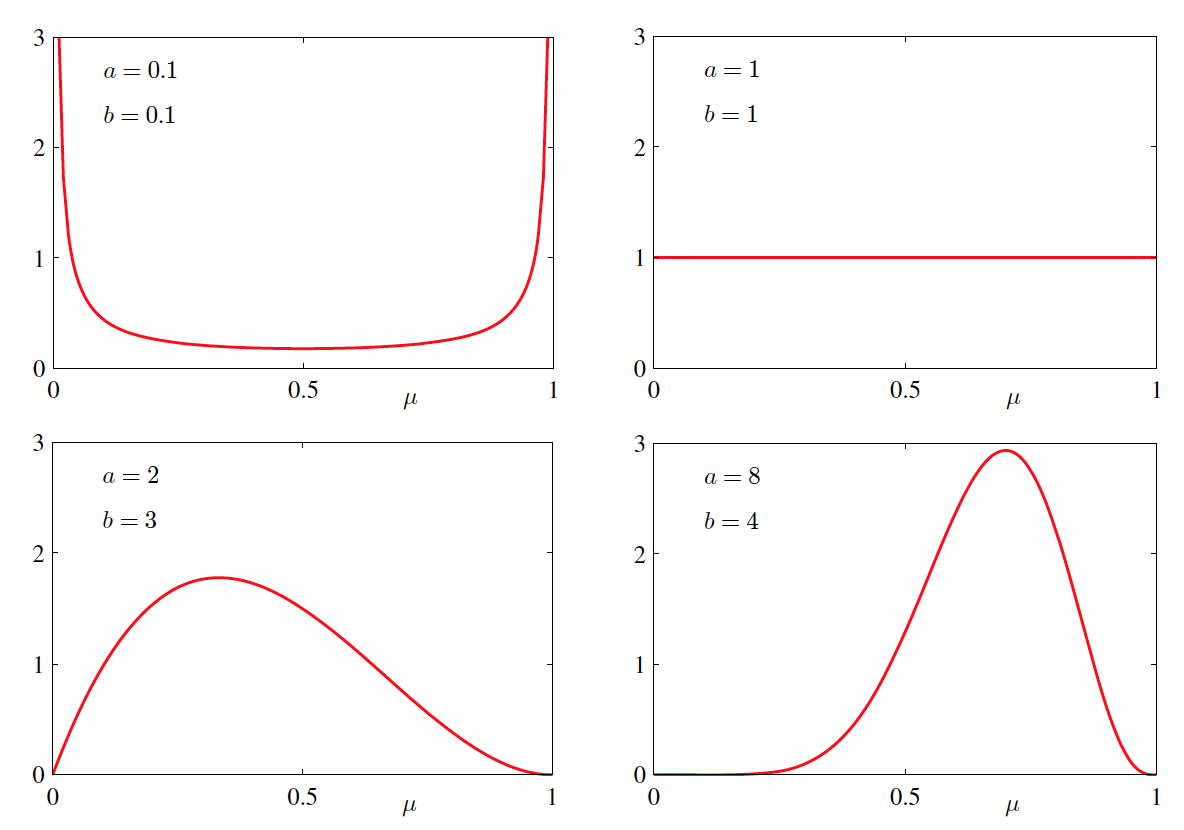Beta 分布的均值和方差分别有下面两式给出
E[θ]var[θ]=aa+b=ab(a+b)2(a+b+1)\begin{align*}
E[\theta]&=\frac{a}{a+b}\\
var[\theta]&=\frac{ab}{(a+b)^2(a+b+1)}
\end{align*}

共轭分布

我们还是从一个例子讲起。假如你有一个硬币，它有可能是不均匀的，所以投这个硬币有 θ$\theta$ 的概率抛出Head，有 (1−θ)$(1-\theta)$ 的概率抛出Tail。如果抛了五次这个硬币，有三次是Head，有两次是Tail，这个 θ$\theta$ 最有可能是多少呢？如果你必须给出一个确定的值，并且你完全根据目前观测的结果来估计 θ$\theta$，那么显然你会得出结论 θ=35$\displaystyle \theta = \frac{3}{5}$。

但上面这种点估计的方法显然有漏洞，这种漏洞主要体现在实验次数比较少的时候，所得出的点估计结果可能有较大偏差。大数定理也告诉我们，在重复实验中，随着实验次数的增加，事件发生的频率才趋于一个稳定值。一个比较极端的例子是，如果你抛出五次硬币，全部都是Head。那么按照之前的逻辑，你将估计 θ$\theta$ 的值等于 1。也就是说，你估计这枚硬币不管怎么投，都朝上！但是按正常思维推理，我们显然不太会相信世界上有这么厉害的硬币，显然硬币还是有一定可能抛出Tail的。就算观测到再多次的Head，抛出Tail的概率还是不可能为0。

前面介绍的贝叶斯定理或许可以帮助我们。在贝叶斯学派看来，参数 θ$\theta$ 不再是一个固定的值了，而是满足一定的概率分布！回想一下前面介绍的先验概率和后验概率。在估计 θ$\theta$ 时，我们心中可能有一个根据经验的估计，即先验概率，P(θ)$P(\theta)$。而给定一系列实验观察结果 X$X$ 的条件下，我们可以得到后验概率为
P(θ|X)=P(X|θ)P(θ)P(X)P(\theta|X)=\frac{P(X|\theta)P(\theta)}{P(X)}
在上面的贝叶斯公式中，P(θ)$P(\theta)$ 就是个概率分布。这个概率分布可以是任何概率分布，比如高斯分布，或者刚刚提过的 Beta 分布。下图是Beta(5,2)的概率分布图。如果我们将这个概率分布作为 P(θ)$P(\theta)$，那么我们在还未抛硬币前，便认为 θ$\theta$ 很可能接近于0.8，而不大可能是个很小的值或是一个很大的值。换言之，我们在抛硬币前，便估计这枚硬币更可能有0.8的概率抛出正面。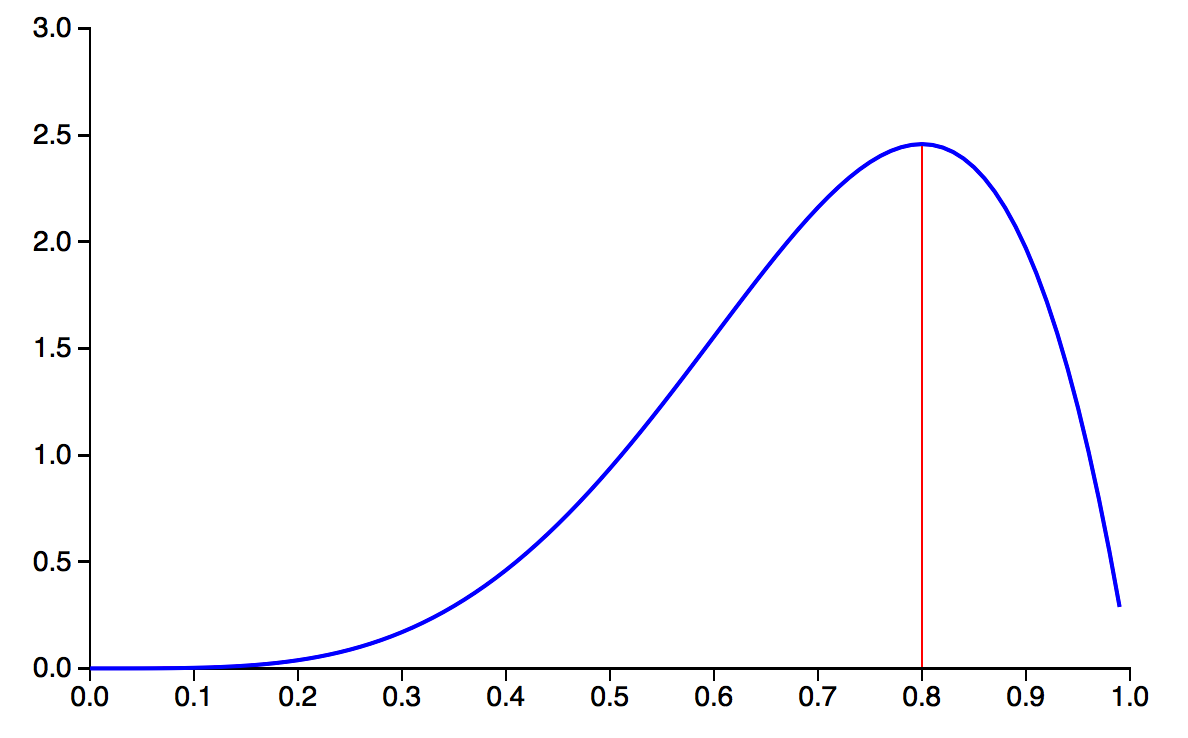虽然 P(θ)$P(\theta)$ 可以是任何种类的概率分布，但是如果使用Beta 分布，会让之后的计算更加方便。我们接着继续看便知道这是为什么了。况且，通过调节 Beta 分布中的 a$a$ 和 b$b$，你可以让这个概率分布变成各种你想要的形状！Beta 分布已经很足够表达我们事先对 θ$\theta$ 的估计了。

现在我们已经估计好了 P(θ)$P(\theta)$ 为一个 Beta 分布，那么 P(X|θ)$P(X|\theta)$ 是多少呢？其实就是个二项（Binomial）分布。继续以前面抛5次硬币抛出3次Head的观察结果为例，X=抛5次硬币3次结果为Head的事件$X=抛5次硬币3次结果为Head的事件$， 则 P(X|θ)=C52θ3(1−θ)2$P(X|\theta)=C_2^5\theta^3(1-\theta)^2$。

贝叶斯公式中分母上的 P(X)$P(X)$ 是个Normalizer，或者叫做边缘概率。在 θ$\theta$ 是离散的情况下，P(X)$P(X)$ 就是 θ$\theta$ 为不同值的时候，P(X|θ)$P(X|\theta)$ 的求和。例如，假设我们事先估计硬币抛出正面的概率只可能是0.5或者0.8，那么 P(X)=P(X|θ=0.5)+P(X|θ=0.8)$P(X) = P(X|\theta=0.5)+P(X|\theta=0.8)$，计算时分别将 θ=0.5$\theta=0.5$ 和 θ=0.8$\theta=0.8$ 代入到前面的二项分布公式中。而如果我们采用 Beta 分布，θ$\theta$的概率分布在[0,1]之间是连续的，所以要用积分，即
P(X)=∫10P(X|θ)P(θ)dθP(X)=\int_0^1P(X|\theta)P(\theta)d\theta
下面的证明就告诉我们：P(θ)$P(\theta)$ 是个 Beta 分布，那么在观测到“X=抛5次硬币中出现3个head$X=抛5次硬币中出现3个head$”的事件后，P(θ|X)$P(\theta|X)$ 依旧是个 Beta 分布！只是这个概率分布的形状因为观测的事件而发生了变化。
P(θ|X)=P(X|θ)P(θ)P(X)=P(X|θ)P(θ)∫10P(X|θ)P(θ)dθ=C52θ3(1−θ)21B(a,b)θa−1(1−θ)b−1∫10C52θ3(1−θ)21B(a,b)θa−1(1−θ)b−1dθ=θ(a+3−1)(1−θ)(b+2−1)∫10θ(a+3−1)(1−θ)(b+2−1)dθ=θ(a+3−1)(1−θ)(b+2−1)B(a+3,b+2)=Beta(θ|a+3,b+2)\begin{align}
P(\theta|X) &= \frac{P(X|\theta)P(\theta)}{P(X)}
=\frac{P(X|\theta)P(\theta)}{\int_0^1P(X|\theta)P(\theta)d\theta}\\
&=\frac{C_2^5\theta^3(1-\theta)^2\frac{1}{\mathbf{B}(a,b)}\theta^{a-1}(1-\theta)^{b-1}}{\int_0^1C_2^5\theta^3(1-\theta)^2\frac{1}{\mathbf{B}(a,b)}\theta^{a-1}(1-\theta)^{b-1} d\theta}\\
&=\frac{\theta^{(a+3-1)}(1-\theta)^{(b+2-1)}}{\int_0^1 \theta^{(a+3-1)}(1-\theta)^{(b+2-1)} d\theta}\\
&=\frac{\theta^{(a+3-1)}(1-\theta)^{(b+2-1)}}{\mathbf{B}(a+3,b+2)}\\
&=Beta(\theta | a+3, b+2)
\end{align}

因为观测前后，对 θ$\theta$ 估计的概率分布均为 Beta 分布，这就是为什么使用 Beta 分布方便我们计算的原因了。当我们得知 P(θ|X)=Beta(θ|a+3,b+2)$P(\theta|X)=Beta(\theta|a+3, b+2)$后，我们就只要根据 Beta 分布的特性，得出 θ$\theta$ 最有可能等于多少了。（即 θ$\theta$ 等于多少时，观测后得到的 Beta 分布有最大的概率密度）。

例如下图，仔细观察新得到的 Beta 分布，和上一图中的概率分布对比，发现峰值从0.8左右的位置移向了0.7左右的位置。这是因为新观测到的数据中，5次有3次是head（60%），这让我们觉得，θ$\theta$ 没有0.8那么高。但由于我们之前觉得 θ$\theta$ 有0.8那么高，我们觉得抛出head的概率肯定又要比60%高一些！这就是Bayesian方法和普通的统计方法不同的地方。我们结合自己的先验概率和观测结果来给出预测。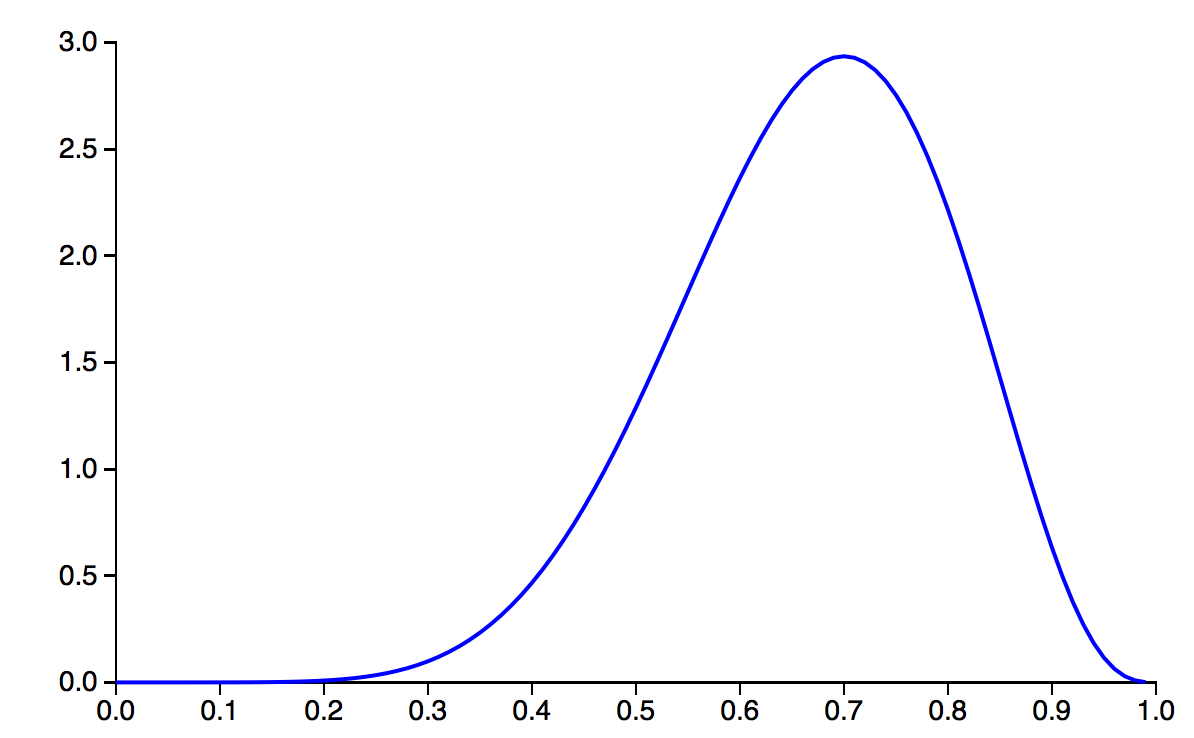如果我们投的不是硬币，而是一个多面体（比如骰子），那么我们就要使用 Dirichlet 分布了。使用Dirichlet 分布之目的，也是为了让观测后得到的posterior probability依旧是 Dirichlet 分布。关于 Dirichlet 分布的话题我们会在后续的文章中继续介绍。

到此为止，我们终于可以引出“共轭性”的概念了！后验概率分布（正⽐于先验和似然函数的乘积）拥有与先验分布相同的函数形式。这个性质被叫做共轭性（Conjugacy）。共轭先验（conjugate prior）有着很重要的作⽤。它使得后验概率分布的函数形式与先验概率相同，因此使得贝叶斯分析得到了极⼤的简化。例如，二项分布的参数之共轭先验就是我们前面介绍的 Beta 分布。多项式分布的参数之共轭先验则是 Dirichlet 分布，⽽⾼斯分布的均值之共轭先验是另⼀个⾼斯分布。

总的来说，对于给定的概率分布 P(X|θ)$P(X|\theta)$，我们可以寻求一个与该似然函数 ，即P(X|θ)$P(X|\theta)$， 共轭的先验分布 P(θ)$P(\theta)$，如此一来后验分布 P(θ|X)$P(\theta|X)$ 就会同先验分布具有相同的函数形式。而且对于任何指数族成员来说，都存在有一个共轭先验。

参考文献

 以上内容部分引自“胖胖小龟宝”在http://bbs.pinggu.org/上的帖子

 Pattern Recognition And Machine Learning,  Christopher Bishop

 抛硬币的例子来自http://maider.blog.sohu.com/306392863.html
展开全文先验概率 Beta分布 Gamma函数 狄利克雷分布
• 针对贝叶斯网络后验概率需计算样本边际分布, 计算代价大的问题, 将共轭先验分布思想引入贝叶斯分类, 提出了基于共轭先验分布的贝叶斯网络分类模型. 针对非区间离散样本, 提出一种自适应的样本离散方法, 将小波包...
• beta分布是伯努利分布和二项式分布的共轭先验 狄利克雷分布是多项式的共轭分布 http://www.johndcook.com/blog/conjugate_prior_diagram/#beta这个链接里共轭分布的图
• 文章目录一、共轭先验分布定义二、正态均值的共轭先验分布1.公式理论2.Example: Normal mean3.R code 一、共轭先验分布定义 设 π(θ)\pi(\theta)π(θ) 是 θ\thetaθ 的先验密度，假设由抽样信息算得的后验密度...朴素贝叶斯算法 r语言
• 在模型参数估计中，根据贝叶斯方法，已知参数的prior和likelihood，可以求得参数的posterior，我们通常希望先验和后验是共轭的，但为什么我们要采用共轭先验呢？我们在这里首先考虑 prior 的不同形式的区别。 考虑...
• 在贝叶斯统计中，如果后验分布与先验分布属于同类，则先验分布与后验分布被称为共轭分布，而先验分布被称为似然函数的共轭先验。比如，高斯分布家族在高斯似然函数下与其自身共轭 (自共轭)。这个概念，以及"共...
• ConjugatePriors.jl：一个Julia包，用于支持共轭先验分布
• 如果你读过贝叶斯学习方面的书或者论文，想必是知道共轭先验这个名词的。 现在假设你闭上眼睛，你能准确地说出共轭分布是指哪个分布和哪个分布式共轭的吗？ 我之前就常常把这个关系弄错，现在记录如下，以加强印象...
• 理解共轭先验对于应用贝叶斯理论的人来说，当你观察一个事件x ,你预估计并给出其内部参数θ ，表示你对于事件x 发生的置信程度。如果你熟悉贝叶斯方法，当你每次观测到新的x 数据时你就会更新你预先给出的参数θ。...计算机视觉
• 如果你读过贝叶斯学习方面的书或者论文，想必是知道共轭先验这个名词的。 贝叶斯学派和频率学派的区别之一是特别重视先验信息对于inference的影响，而引入先验信息的手段有“贝叶斯原则“（即把先验信息当着均匀...
• 第二章一共是两个部分，共轭先验分布与充分统计量。今天就先复习共轭先验分布。我们会介绍一下基本概念，然后说一说课本正文里出现的共轭先验分布以及我作业做错的共轭先验分布，问题主要出在定义域上。大家不要重蹈...
• 1.分布的核正态分布为 ，如果已知分布为正态分布我们只需要知道式子的一部分就可以推出分布，如 ， 为正态分布，根据正态分布的形式，只要知道 就可以计算出 。...共轭先验分布贝叶斯定理， 左边是一个关...beta分布 gamma分布
• 贝叶斯学派和频率学派的区别之一是特别重视先验信息对于inference的影响，而引入先验信息的手段有“贝叶斯原则“（即把先验信息当着均匀分布）等四大类其中有重要影响的一类是：共轭先验现在假设我...
• 如果你读过贝叶斯学习方面的书或者论文，想必是知道共轭先验这个名词的。 贝叶斯学派和频率学派的区别之一是特别重视先验信息对于inference的影响，而引入先验信息的手段有“贝叶斯原则“（即把先验信息当着均匀...
• 读数理统计学导论时，遇到过共轭先验的概念。 贝叶斯判别准则中，分别假设了先验分布p(θ)p(\theta)，后验分布p(θ|X)p(\theta|X)，以及p(X),p(X|θ)p(X), p(X|\theta)似然函数。贝叶斯定理可以写作：P(θ|X)=P(θ)...统计学 函数...